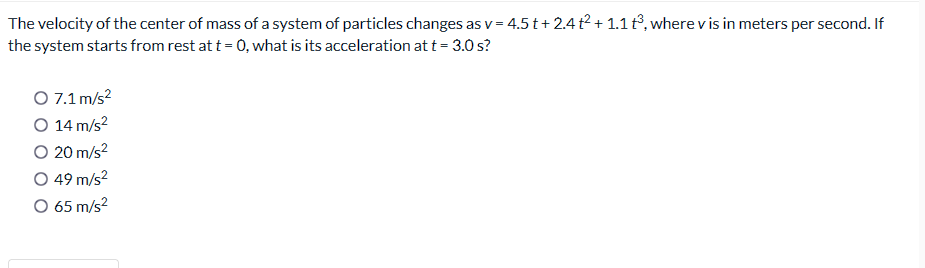# Question The velocity of the center of mass of a system of particles changes as $$v=4.5 t+2.4 t^{2}+1.1 t^{3}$$, where $$v$$ is in meters per second. If the system starts from rest at $$t=0$$, what is its acceleration at $$t=3.0 \mathrm{~s}$$ ? $\begin{array}{l} 7.1 \mathrm{~m} / \mathrm{s}^{2} \\ 14 \mathrm{~m} / \mathrm{s}^{2} \\ 20 \mathrm{~m} / The velocity of the center of mass of a system of particles changes as $$v=4.5 t+2.4 t^{2}+1.1 t^{3}$$, where $$v$$ is in meters per second. If the system starts from rest at $$t=0$$, what is its acceleration at $$t=3.0 \mathrm{~s}$$ ? \[ \begin{array}{l} 7.1 \mathrm{~m} / \mathrm{s}^{2} \\ 14 \mathrm{~m} / \mathrm{s}^{2} \\ 20 \mathrm{~m} / \mathrm{s}^{2} \\ 49 \mathrm{~m} / \mathrm{s}^{2} \\ 65 \mathrm{~m} / \mathrm{s}^{2} \end{array}$Transcribed Image Text: The velocity of the center of mass of a system of particles changes as $$v=4.5 t+2.4 t^{2}+1.1 t^{3}$$, where $$v$$ is in meters per second. If the system starts from rest at $$t=0$$, what is its acceleration at $$t=3.0 \mathrm{~s}$$ ? $\begin{array}{l} 7.1 \mathrm{~m} / \mathrm{s}^{2} \\ 14 \mathrm{~m} / \mathrm{s}^{2} \\ 20 \mathrm{~m} / \mathrm{s}^{2} \\ 49 \mathrm{~m} / \mathrm{s}^{2} \\ 65 \mathrm{~m} / \mathrm{s}^{2} \end{array}$
Transcribed Image Text: The velocity of the center of mass of a system of particles changes as $$v=4.5 t+2.4 t^{2}+1.1 t^{3}$$, where $$v$$ is in meters per second. If the system starts from rest at $$t=0$$, what is its acceleration at $$t=3.0 \mathrm{~s}$$ ? $\begin{array}{l} 7.1 \mathrm{~m} / \mathrm{s}^{2} \\ 14 \mathrm{~m} / \mathrm{s}^{2} \\ 20 \mathrm{~m} / \mathrm{s}^{2} \\ 49 \mathrm{~m} / \mathrm{s}^{2} \\ 65 \mathrm{~m} / \mathrm{s}^{2} \end{array}$&#12304;General guidance&#12305;The answer provided below has been developed in a clear step by step manner.Step1/3Given \begin{align*} \mathrm{{v}} &= \mathrm{{4.5}{t}+{2.4}{t}^{{{2}}}+{1.1}{t}^{{{3}}}} \end{align*}we have to calculate acceleration at t = 3.0 s Explanation:here of use differentiation to find acceleration at 3 s .Step2/3since acceleration: change in velocity with respect to time i.e a = dv/dt\( \begin{align*} \mathrm{{a}} &= \mathrm{\frac{{{d}{\left({4.5}{t}+{2.4}{t}^{{{2}}}+{1.1}{t}^{{{3}}}\right)}}}{{{\left.{d}{t}\right.}}}} \\[3pt]\mathrm{{a}} &= \ ... See the full answer